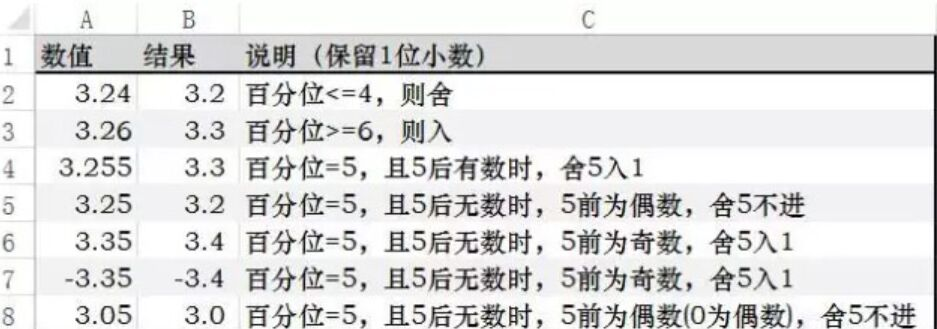# 在表达式中对数字取整或保留指定位小数

CL 2021/9/16 发布 · 2022/2/10 更新 · 883 次阅读

# 取整

--------

C#取整函数使用详解：
1、Math.Round是"就近舍入"，当要舍入的是5时与"四舍五入"不同(取偶数)，如：
Math.Round(0.5,0)=0
Math.Round(1.5,0)=2
Math.Round(2.5,0)=2
Math.Round(3.5,0)=4

2、Math.Truncate 计算双精度浮点数的整数部分，即直接取整数，如：
Math.Truncate(-123.55)=-123,
Math.Truncate(123.55)=123

3、Math.Ceiling 取天板值，即向上取整，与"四舍五入"无关。
Math.Ceiling(1) = 1
Math.Ceiling(1.1) = 2
Math.Ceiling(1.5) = 2
Math.Ceiling(3.1) = 4

4、Math.Floor 取地板值，即向下取整，与"四舍五入"无关。
Math.Floor(1) = 1
Math.Floor(1.1) = 1
Math.Floor(1.5) = 1
Math.Floor(3.9) = 3

int a = 5; int b = 2;   lbl.Text = Convert.ToString(Math.Ceiling((double)a / (double)b));

# 保留指定位小数

Math.Round(原始数字,  保留位数)• Math.Round( 1.2345, 2)  = 1.23
• Math.Round( 1.2350, 2) = 1.24
• Math.Round( 1.2, 2) = 1.2   （这里是数字，对数字来说1.20 = 1.2）

## 转化为文本

• {数字变量}.ToString("F2")  = "5678.12"
• {数字变量}.ToString("F2")  = "56789.1234"
• {数字变量}.ToString("F6")  = "56789.123450"
• {数字变量}.ToString("N2")  = "56,789.12"
• {数字变量}.ToString("N4")  = "56,789.1234"
• {数字变量}.ToString("P2")  = "5,678,912.34%"
• {数字变量}.ToString("#0.00") = "56789.12"

· {{comment.createTimeStr}}